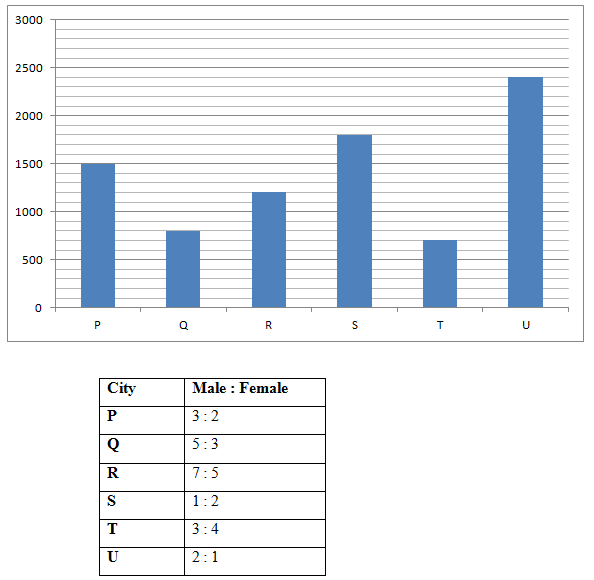# NIACL AO Prelims – Quantitative Aptitude Questions Day- 69

Dear Readers, Bank Exam Race for the Year 2019 is already started, To enrich your preparation here we have providing new series of Practice Questions on Quantitative Aptitude – Section. Candidates those who are preparing for NIACL AO Prelims 2019 Exams can practice these questions daily and make your preparation effective.

[WpProQuiz 4780]

Directions (Q. 1 – 5): What value should come in place of question mark (?) in the following number series?

1) 12, 20, 67, ? , 1185

a) 327

b) 289

c) 265

d) 313

e) 358

2) 6336, 3168, 2112, 1584, 1267.2, ?

a) 1056

b) 1124

c) 1338

d) 1452

e) 1544

3) 21, 43, 175, 1055, ?

a) 7548

b) 7924

c) 8135

d) 8447

e) 8562

4) 1263, 1390, 1617, 1925, 2297, ?

a) 2564

b) 2718

c) 2345

d) 2976

e) 2852

5) 762, 758, 748, 720, 638, ?

a) 452

b) 428

c) 394

d) 375

e) 436

Directions (Q. 6 – 10) Study the following information carefully and answer the given questions:

The following bar graph shows the total number of people got loan from different banks in a certain city and the table shows the ratio of male and female among them.6) Find the difference between the total number of males got loan from bank P and R together to that of total number of people got loan from bank S?

a) 350

b) 550

c) 200

d) 700

e) None of these

7) Find the ratio between the total number of females got loan from S and U together to that of total number of males got loan from P and Q together?

a) 12: 5

b) 10: 7

c) 15: 8

d) 17: 9

e) None of these

8) Find the total number of females got loan from bank Q, S and T together?

a) 2300

b) 2700

c) 2100

d) 1900

e) None of these

9)  Total number of people got loan from P, R and T together is what percentage of total number of people got loan from Q, S and U together?

a) 68 %

b) 54 %

c) 77 %

d) 86 %

e) None of these

10) Total number of males got loan from S and U together is what percentage more/less than the total number of females got loan from P and T together?

a) 140 % more

b) 140 % less

c) 120 % more

d) 120 % less

e) None of these

Directions (Q. 1 – 5):

The pattern is, *1 + 23, *2 + 33, *3 + 43, *4 + 53,…

The pattern is, *1/2, *2/3, *3/4, *4/5, *5/6,…

The pattern is, *2 + 1, *4 + 3, *6 + 5, *8 + 7,…

1263,               1390,               1617,               1925,               2297,               2718,

127                  227                  308                  372                  421

100                  81                    64                    49

(102)                (92)                  (82)                  (72)

The difference of difference is, 102, 92, 82, 72, 62,…

The pattern is, – (31 +1), – (32 +1), – (33 +1), – (34 +1), – (35 +1),…

Directions (Q. 6 – 10)

The total number of males got loan from bank P and R together

= > 1500*(3/5) + 1200*(7/12)

= > 900 + 700 = 1600

The total number of people got loan from bank S = 1800

Required difference = 1800 – 1600 = 200

The total number of females got loan from S and U together

= > 1800*(2/3) + 2400*(1/3)

= > 1200 + 800 = 2000

The total number of males got loan from P and Q together

= > 1500*(3/5) + 800*(5/8)

= > 900 + 500 = 1400

Required ratio = 2000: 1400 = 10: 7

The total number of females got loan from bank Q, S and T together

= > 800*(3/8) + 1800*(2/3) + 700*(4/7)

= > 300 + 1200 + 400 = 1900

Total number of people got loan from P, R and T together

= > 1500 + 1200 + 700 = 3400

Total number of people got loan from Q, S and U together

= > 800 + 1800 + 2400 = 5000

Required % = (3400/5000)*100 = 68 %

The total number of males got loan from S and U together

= > 1800*(1/3) + 2400*(2/3)

= > 600 + 1600 = 2200

The total number of females got loan from P and T together

= > 1500*(2/5) + 700*(4/7)

= > 600 + 400 = 1000

Required % = [(2200 – 1000)/1000]*100 = 120 % more